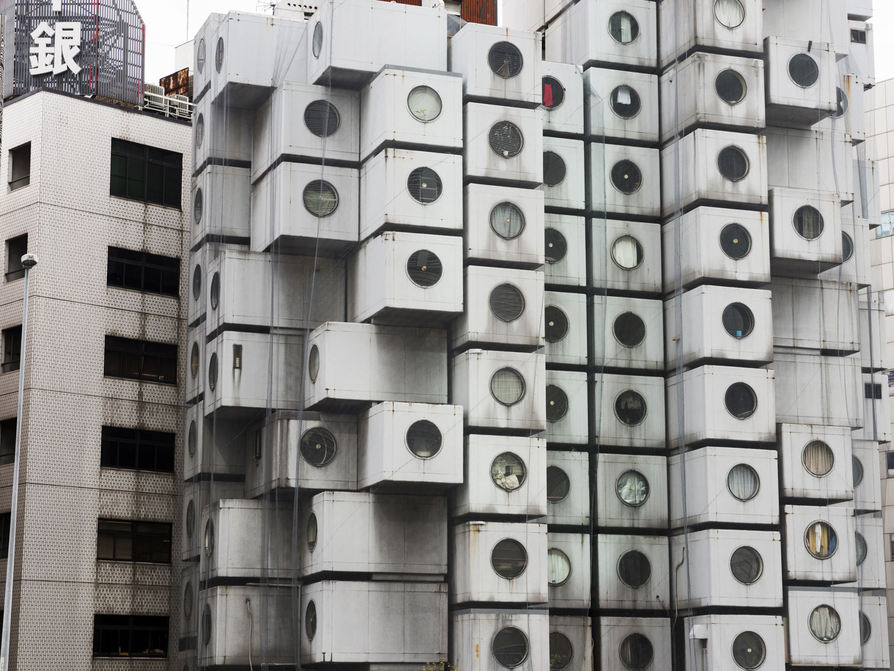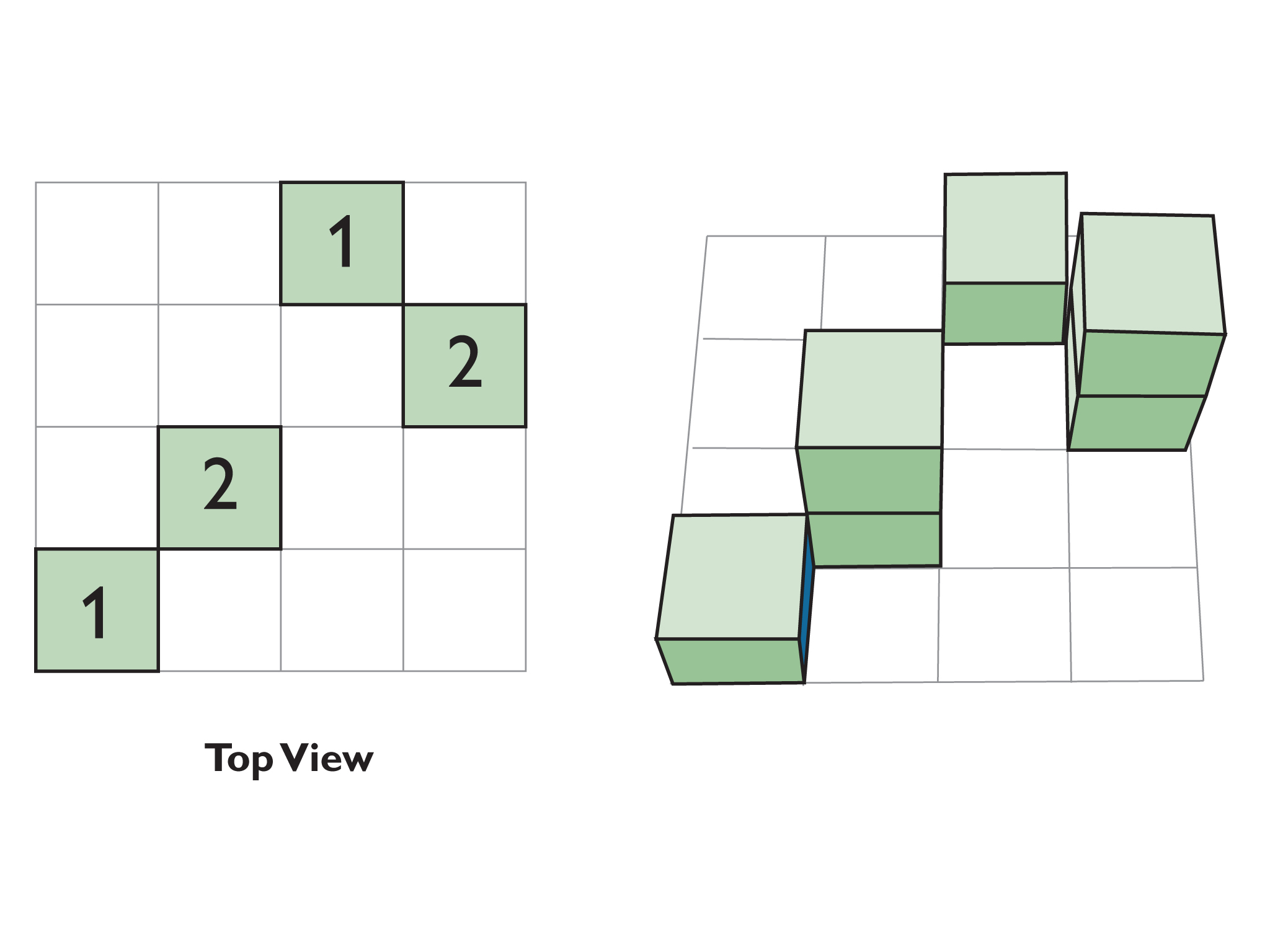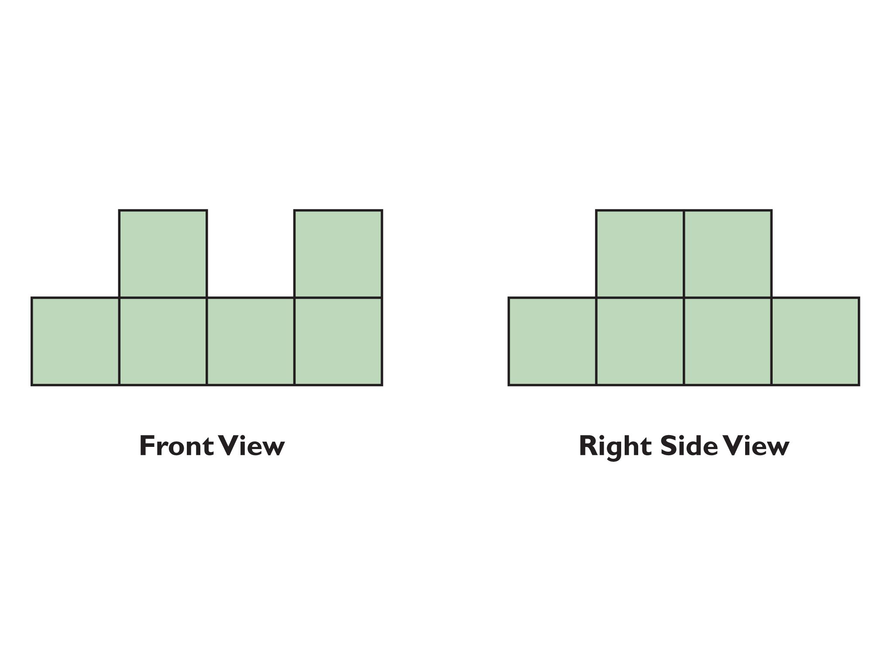Subject:
Geometry
Material Type:
Lesson Plan
Level:
Middle School
7
Provider:
Pearson
Tags:
• Cube Structure
Language:
English
Media Formats:
Interactive, Text/HTML

# Solving Problems That Require Spatial Reasoning## Overview

Students are presented with a front view and a side view of a cube structure. They use spatial reasoning to picture what the entire structure looks like and to determine the least number of cubes they would need to build the structure.

# Key Concepts

Students are expected to use the mathematical skills they have acquired in previous lessons or in previous math courses. The lessons in this unit focus on developing and refining problem-solving skills. Students will:

• Try a variety of strategies to approaching different types of problems.
• Devise a problem-solving plan and implement their plan systematically.
• Become aware that problems can be solved in more than one way.
• See the value of approaching problems in a systematic manner.
• Communicate their approaches with precision and articulate why their strategies and solutions are reasonable.
• Make connections between previous learning and real-world problems.
• Create efficacy and confidence in solving challenging problems in a real-world setting.

# Goals and Learning Objectives

• Visualize three-dimensional spaces.
• Solve problems that require spatial reasoning.
• Design and implement a problem-solving plan.
• Articulate strategies, thought processes, and approaches to solving a problem and defend why the solution is reasonable.

# Lesson Guide

The purpose of the opening is to elicit thought-provoking conversations between the students and engage them in the problem situation. You want to pique students’ interest in the problem as well as invoke their prior knowledge of the mathematics needed to solve the problem.

Tell students that today they will solve a problem that requires them to visualize a three-dimensional object without seeing the whole object.

Have students discuss the question with a partner for 1 to 2 minutes. Then discuss the question as a class. Elicit the point that it is difficult to count the number of capsules when you can see only one side of the building.

Focus on engaging students in the context of the problem rather than on the specific mathematics involved. Use the work period and the class discussion to focus on the mathematical strategies that students used.

ELL: Give students background knowledge about the Nakajin Capsule Tower. Students should know that the tower was built in Tokyo, Japan in 1972. Also, discuss why a building like this was needed in Japan. Discuss population density and sustainability as reasons why the building was constructed in this style.

# Tower

Kisho Kurokawa designed this tower built of capsules. It is called the Nakagin Capsule Tower and was built in Japan in 1972.

• How many capsules do you think are in the tower?# Lesson Guide

Demonstrate how to use the Block Builder interactive.

# Block Builder

Your teacher will demonstrate how to use the Block Builder interactive.

INTERACTIVE: Block Builder

# Lesson Guide

Discuss the Math Mission. Students will build an arrangement of cubes when given only the front view and side view of the arrangement.

SWD: Provide blocks or snap cubes for students. When students work with manipulatives, they are using their visual and tactile skills to enhance their learning experience. Manipulatives make learning easier and are also fun to work with. Some students may enjoy working with manipulatives over the Block Builder interactive.

## Opening

Build an arrangement of cubes when given the front view and side view of the arrangement.

# Lesson Guide

Have students work on this problem independently for a few minutes to organize their thoughts as well as the information provided in the problem. During independent time, encourage students to devise and implement a plan for solving the problem. Refrain from guiding students’ thinking and providing hints, and give them this opportunity to truly work independently.

Then, have students work with partners. Ask students to share the approaches and solutions they generated during independent time. Have partners agree on a problem-solving plan to implement together as they solve the remainder of the problem. They may also compare and refine their solutions together to come to a consensus on the correct answer to the problem. Carefully monitor students for understanding, and ask probing questions. Select students to share their responses during the class discussion. Provide students with blocks, if they choose, to help them solve the problem.

# Mathematics

Students work independently and with a partner to explore the least number of blocks needed to complete a structure. It is essential for students to articulate their thought processes as they create and implement their problem-solving plan. Highlighting these thought processes builds confidence and understanding as students work through this problem-solving unit.

Discuss the essential steps in creating and implementing a problem-solving plan:

1. Understand the situation.
2. Represent the situation.
5. Prepare a presentation.
6. Use your analysis to solve other problems.

An important first step is to understand the problem situation. Ask students how this problem is different from the beginning activity in which they had to try to guess the number of cubes from looking at just one side of the building. Discuss as a class how students have to determine the least number of cubes needed to construct the figure given a front view and a side view.

SWD: Students with disabilities may have difficulty interpreting the visual information. Provide students with tactile 3-D manipulatives.

# Mathematical Practices

Mathematical Practice 1: Make sense of problems and persevere in solving them.

Students organize the information shown in the two views of the building and make sense of how a structure may have these two different views. They then begin creating a systematic way to find the total number of cubes in the structure and persevere until they have found the fewest number of cubes that work.

Mathematical Practice 2: Reason abstractly and quantitatively.

Students use abstract reasoning to make connections between the views of the building and the cubes needed to create these views. They then use their quantitative reasoning to find the fewest number of cubes needed for the structure.

Mathematical Practice 3: Construct viable arguments and critique the reasoning of others.

Students defend why they think their solution is reasonable and have the opportunity to critique the thinking of others during the class discussion.

Mathematical Practice 4: Model with mathematics.

Students build tangible or virtual models of the structure to show how their building matches the views given in the problem.

Mathematical Practice 5: Use appropriate tools strategically.

Students use the Block Builder interactive, actual blocks, or pencil and paper to prepare their solutions.

Mathematical Practice 6: Attend to precision.

Students attend to the precision in the problem by ensuring their structure matches the views given in the problem.

Mathematical Practice 7: Look for and make use of structure.

Students make use of the problem-solving process as a structure in approaching and solving the problem.

# Interventions

Student has difficulty getting started.

• What do you know about the problem?
• What are you trying to find?
• What strategies can you try?

Student has a solution, but it is incorrect.

• How can you use fewer cubes in your structure?
• Which cubes are essential and which are unnecessary?
• Does your structure match the front and side views given in the problem?

Student has a solution but is having a hard time articulating their reasoning.

• How can you explain your thinking to others?
• Can you restate your solution in a sentence?

Student has a solution and is waiting on others to finish.

• Explain your strategy for solving the problem.
• Why did you approach the problem that way?
• Can you use a different method?
• What are some strategies you tried that did not work? Why didn’t they work?
• Is there more than one solution to this problem? Why or why not?

• 6 cubes
• Possible solution (looking from top view with numbers indicating number of cubes in the stack):# Cubes

The figures show the front and right side views of a construction made of cubes.

Use the Block Builder interactive, actual blocks, or paper and pencil to complete the following tasks.

• What is the least number of cubes that you could use to make a cube structure that has a front view and right side view as shown above?
• Sketch a diagram by hand, use blocks, or use the Block Builder interactive.

You can start by building the bottom layer first. Look at the structure from the front: how many cubes do you need based on this view?

Look at the structure from the side: how many cubes do you need based on this view?

If you have difficulty getting started, try this describing the task in your own words to your partner.

• What do you know?
• What are you trying to find?
• What strategy can you try?

If you have a solution, try this:

• Explain your strategy for solving the problem.
• Why did you approach the problem that way?INTERACTIVE: Block Builder

# Preparing For Ways of Thinking

As students work through the problem, identify students who use different strategies to solve the problem and highlight these strategies during the class discussion.

Look for students who:

• Use the Block Builder interactive to help them find the solution.
• Use blocks to help them find the solution.
• Use too many or too few blocks to create the structure.
• Defend their solution by stating that the cubes must be connected to create a structure.

# Challenge Problem

Answers will vary. Check students’ problems.

# Prepare a Presentation

Prepare a presentation about your solution to the cube problem. What steps did you use to help you solve this problem? Use illustrations and explain your approach to the class.

# Challenge Problem

Now, think of the problem you just solved, and create a new problem that's similar. Then trade with a partner and solve each other's problem.

Remember to include a front and side view of your building. If the left and right side of your building look different, you must include both views.

# Mathematics

Discuss students’ strategies and the validity of their solutions. Highlight any mathematical processes students used. Students should be refining their own thought processes, correcting solutions, and taking notes. Encourage classmates to critique student work during this time.

• How did you approach the problem? What strategies did you use?
• Can you make the structure using fewer cubes?
• How do you know that your building uses the minimum number of cubes? Are there any unnecessary cubes you can remove?
• How did you persevere in finding the solution?
• What was the most challenging aspect of the problem? How did you overcome this challenge?
• Are there multiple solutions to the problem? Why or why not?

Students may give this incorrect building plan (shown below), looking from top view with numbers indicating number of cubes in the stack.

Explore different structures that match the front and side views given, have students describe how to use these structures and remove cubes until they find the minimum number of cubes needed to match the two views.

• Point out any mathematical processes you observed during work time.
• Have students who completed the Challenge Problem share their work.
• As a class, revisit the mathematical goals and outline the steps taken to generate a problem-solving plan.
• Create a poster to post in the classroom or add the problem-solving steps to your Notebook.
• Have students create a work page in their Notebook called Problem-Solving Steps so they can have a record to reference as well.

Problem-Solving Steps

1. Understand the problem situation. What is the problem asking you to find out? What information is provided? What are the quantities that vary? How are the variables related?
2. Represent the situation (using tables, graphs, equations)
4. Check that the mathematical answer makes sense.
6. Use your analysis to solve other problems.

ELL: When selecting a group of students to present in the “Ways of Thinking” section, ensure students present a topic they are confident about. Have students draw diagrams or demonstrate their knowledge in some other way than through verbal language alone.

# Ways of Thinking: Make Connections

Take notes about other classmates' approaches to the cube problem. Think about the problem-solving process you used to find the number of cubes needed to make this structure.